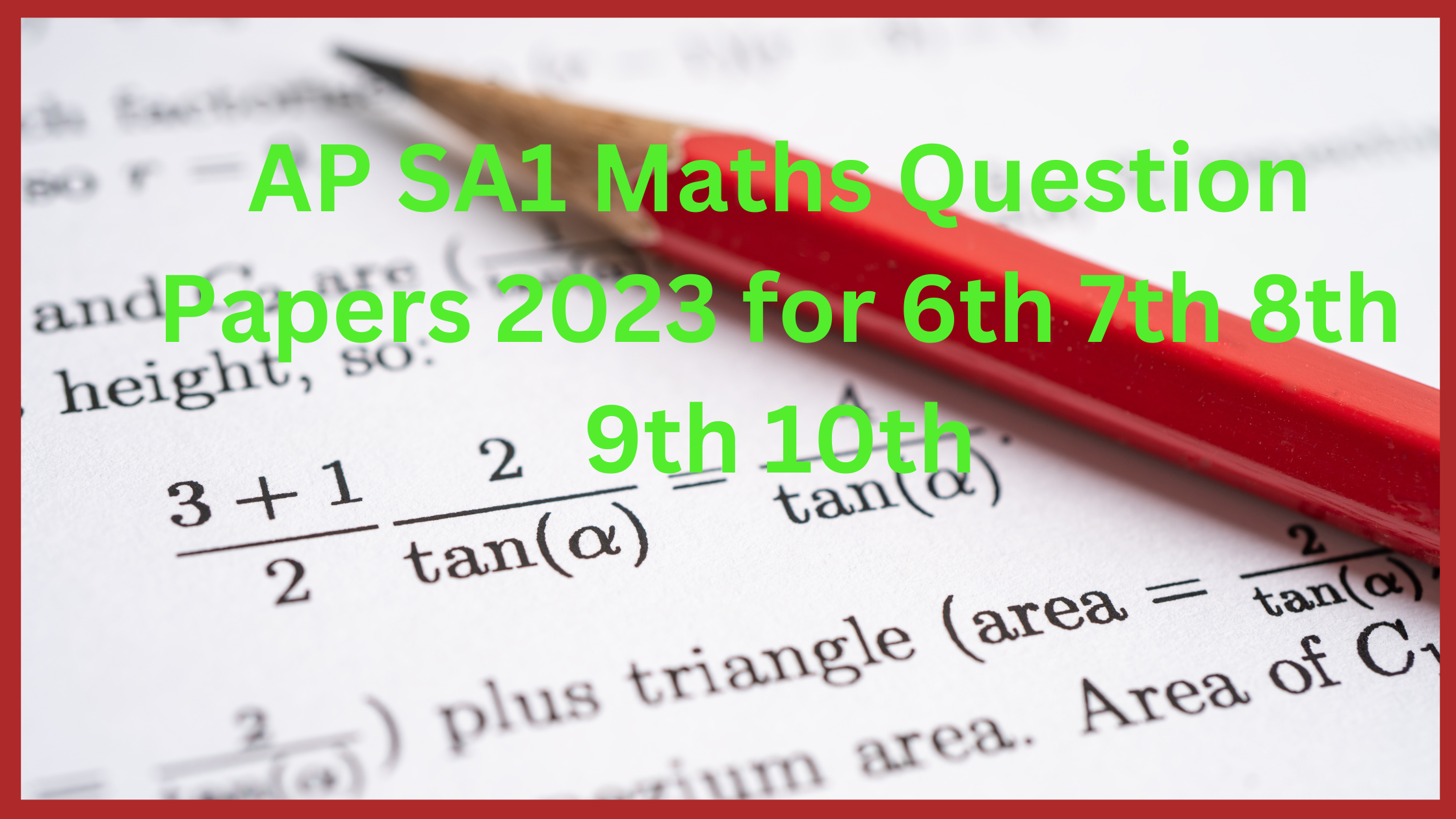# AP SA1 Maths Question Papers 2023 for 6th 7th 8th 9th 10th

AP SA1 Maths Question Papers 2023 for 6th 7th 8th 9th 10th: The education system of the Andhra Pradesh state government follows the Continuous and Comprehensive Evaluation (CCE) pattern of the education system. The textbooks for the children of the AP state are released through Andhra Pradesh State Council for Education Research and Training (APSCERT). The child assessment during the academic period is given based on the Summative Assessment.

What is Summative Assessment?

A summative Assessment is an examination that is conducted twice a year to record the student’s assessment. They are named Summative Assessment – 1 (SA-1) and Summative Assessment – 2 (SA-2). The SA-1 is conducted with half of the syllabus in the middle of the academic year. SA-2 is conducted with the whole syllabus at the end of the year. Based on the performance of the students in SA-1 and SA-2, the students are promoted to the next class.

The SA is for 100 marks, where the exam is conducted for 80 marks and the remaining marks are taken from the Formative Assessments (Internal Assessment) or the project works allotted to the student. The time duration for the Summative exam is 3 hours. The grades are allotted to the students based on the assessment of Summative and it is considered the end of the teaching-learning process.## SA model Question papers / Sa1 Maths Question Paper

The Summative Assessment model papers of classes 5th to 10th are provided by the Andhra Pradesh State SCERT. The Sa1 Maths Question Paper model question paper links are given year-wise for each subject in pdf format. The exam papers will be available in both English and Telugu medium languages at the main website. The before-year examination papers help the students to get good marks and to know the chapters from where the questions are being asked. Every year after the completion of the academic year the question papers in the pdf format are uploaded to the official website.

The previous year’s maths papers for the classes are given below.

Links of 5th, 6th,7th,8th, 9th,10th papers.

### 5th, 6th, 7th, 8th, 9th, 10th Class EM / TM Model Papers and Syllabus

5th Class Maths Syllabus:

• Let’s Recall
• My Number World
• Multiplication and Divisions
• Multiples and Factors
• Geometry
• Data Handling
• Fractions
• Measurements
• Time

6th Class Maths Syllabus:

• Numbers all around us
• Whole numbers
• HCF & LCM
• Integers
• Fractions & Decimals
• Basic Arithmetic
• Introduction to Algebra
• Basic Geometric Concepts
• 2D-3D Shapes
• Practical Geometry
• Perimeter and Area
• Data Handling

7th Class Maths Syllabus:

• Integers
• Fractions & Decimals
• Data Handling
• Simple Equations
• Lines and Angles
• The Triangle and its properties
• Comparing Quantities
• Rational Numbers
• Perimeter and Area
• Algebraic Expressions
• Exponents and Powers
• Symmetry
• Visualising Solid Shapes

8th Class Maths Syllabus:

• Rational Numbers
• Linear Equations in One Variable
• Exponents and Powers
• Comparing Quantities using Proportion
• Square Roots and Cube Roots
• Frequency Distribution Tables and Graphs
• Exploring Geometrical Figures
• Area of Plane Figures
• Direct and Inverse Proportions
• Algebraic Expressions
• Factorisation
• Visualizing 3-D in 2-D
• Surface Area and Volume (Cube-Cuboid)
• Playing with numbers

9th Class Maths Syllabus:

• Number system
• Polynomials
• Co-ordinate Geometry
• Linear Equations in Two variables
• Introduction to Euclid’s Geometry
• Lines and Angles
• Proofs in Mathematics
• Triangles
• Circles
• Heron’s Formula
• Surface Areas and Volumes
• Statistics

10th Class Maths Syllabus:

• Real Numbers
• Sets
• Polynomials
• Pair of Linear Equations in Two Variables
• Progressions
• Co-ordinate Geometry
• Similar Triangles
• Tangents and Secants to a Circle
• Mensuration
• Trigonometry
• Applications of Trigonometry
• Probability
• Statistics